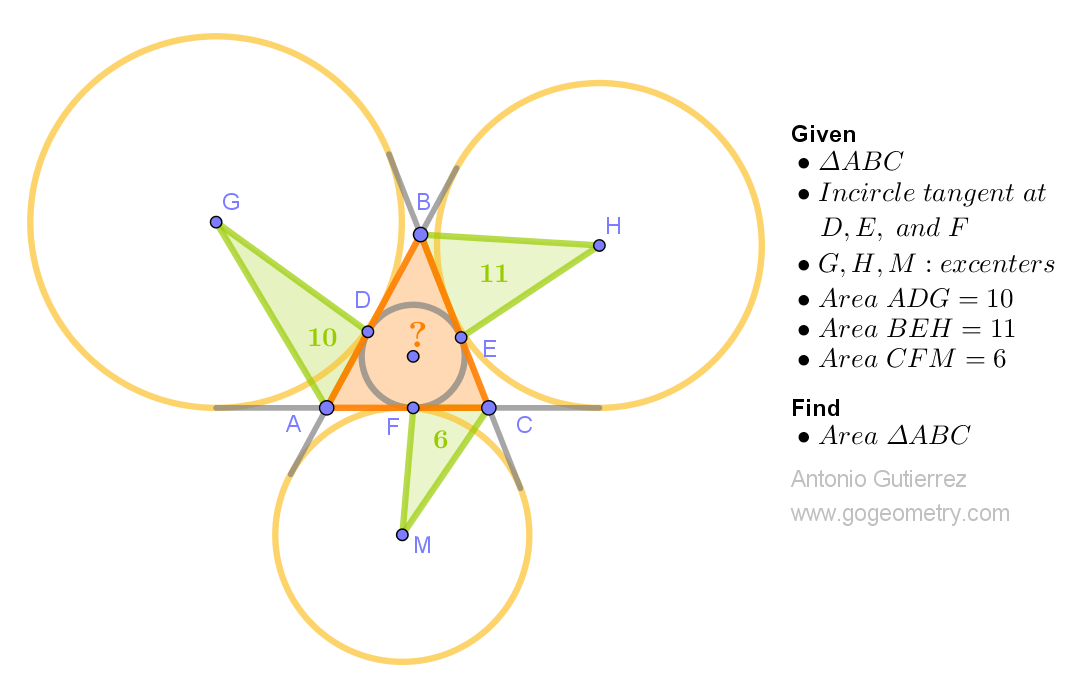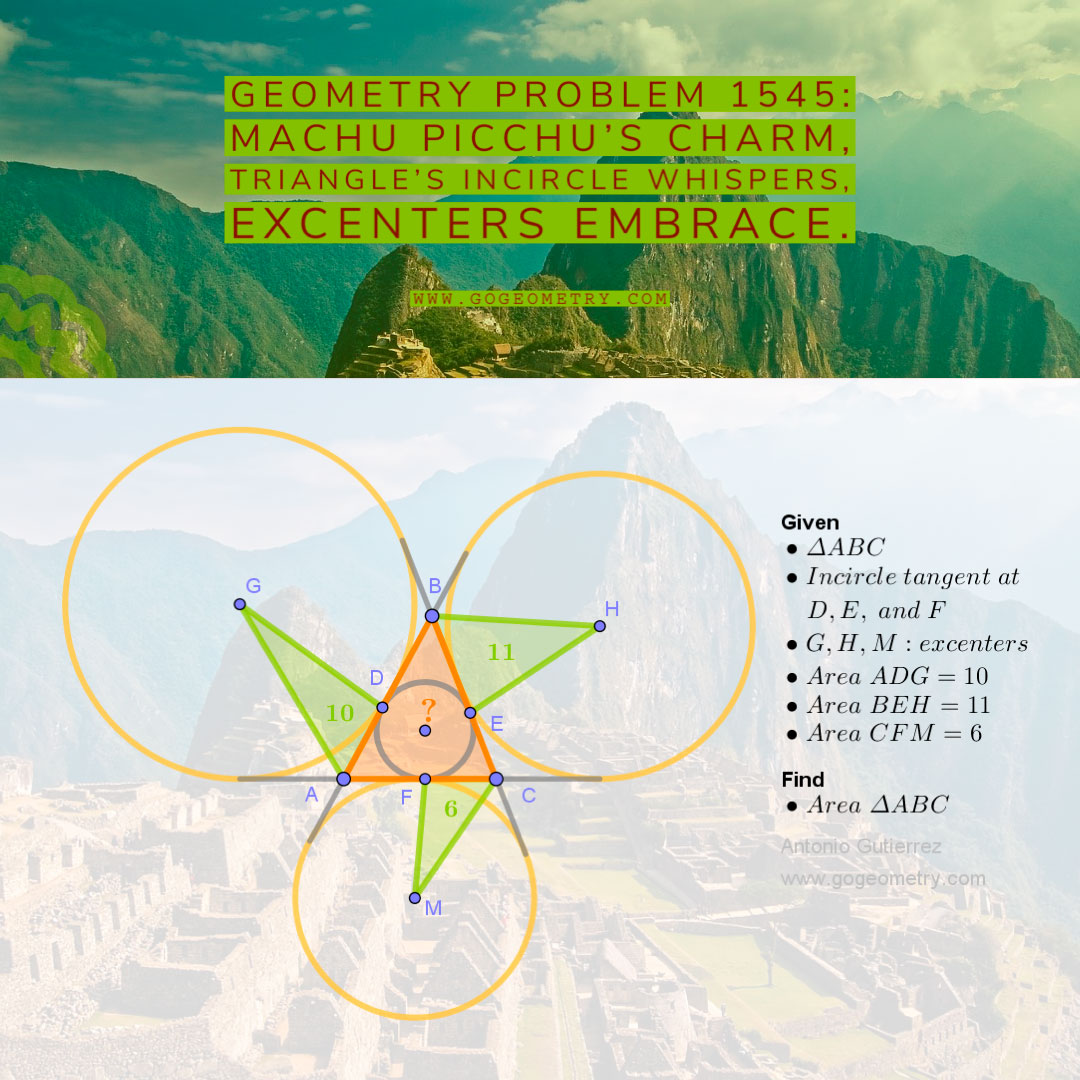# Geometry Problem 1545: Unlock the Geometric Mystery: Calculate the Area of Triangle ABC with Inscribed Circle and Excenters!

Consider triangle ABC with an inscribed circle tangent to the sides AB, BC, and AC at points D, E, and F, respectively. Let G, H, and M denote the excenters relative to sides AB, BC, and AC, respectively. Given that the areas of the triangular regions ADG, BEH, and CFM measure 10, 11, and 6 units, respectively, determine the area of triangle ABC.Inscribed circle's charm,
Area's hidden tale.
With three regions as our guide,
Geometric puzzle unraveled.

## Lines and Shadows: Exploring Problem 1545 with Machu Picchu as the Backdrop